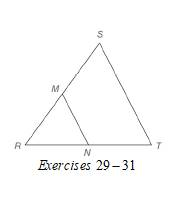Chapter 4.2, Problem 29EElementary Geometry For College St...

7th Edition
Alexander + 2 others
ISBN: 9781337614085

Solutions

Chapter
SectionElementary Geometry For College St...

7th Edition
Alexander + 2 others
ISBN: 9781337614085
Textbook Problem

In Exercises 29 to 31, M and N are the midpoints of sides R S ¯ and R T ¯ of ∆ R S T , respectively. Given: M N = 2 y - 3 S T = 3 y Find: y , M N , and S TTo determine

To find:

y, MN, and ST.

For MN=2y-3

ST=3y

Explanation

Calculation:

Given,

MN=2y-3

ST=3y

M N are the midpoints of sides RS¯ and RT¯ of RST respectively.

A line segment that joins the midpoints of two sides of a triangle is parallel to the third side and has a length equal to one-half the length of the third side.

Thus,

MN=12ST

2y-3=12(3y)

Multiply; by 2 on both sides,

22y-2=2·12(3y)

22y-2=3y

Apply, the distributive property of multiplication over subtraction to the left of the equation

Still sussing out bartleby?

Check out a sample textbook solution.

See a sample solution

The Solution to Your Study Problems

Bartleby provides explanations to thousands of textbook problems written by our experts, many with advanced degrees!

Get Started

In Exercises 1-4, simplify the expression by factoring. x23x28x7

Calculus: An Applied Approach (MindTap Course List)

Find the critical numbers of the function. h(p)=p1p2+4

Single Variable Calculus: Early Transcendentals

True or False: If R is a rectangular region in the plane, then

Study Guide for Stewart's Multivariable Calculus, 8th

Define fraud and explain the safeguards that exist to prevent it.

Research Methods for the Behavioral Sciences (MindTap Course List)